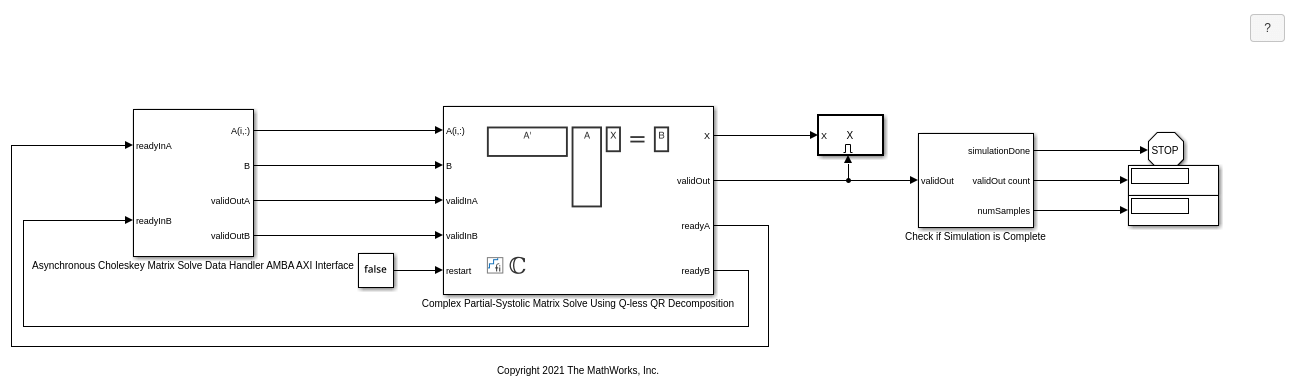# Implement Hardware-Efficient Complex Partial-Systolic Matrix Solve Using Q-less QR Decomposition

This example shows how to implement a hardware-efficient solution to the complex-valued matrix equation A'AX=B using the Complex Partial-Systolic Matrix Solve Using Q-less QR Decomposition block.

### Define Matrix Dimensions

Specify the number of rows in matrix A, the number of columns in matrix A and rows in B, and the number of columns in matrix B.

```m = 300; % Number of rows in A n = 10; % Number of columns in A and rows in B p = 1; % Number of columns in B ```

### Generate Matrices

For this example, use the helper function `complexRandomQlessQRMatrices` to generate random matrices A and B for the problem A'AX=B. The matrices are generated such that the real and imaginary parts of the elements of A and B are between -1 and +1, and A is full rank.

```rng('default') [A,B] = fixed.example.complexRandomQlessQRMatrices(m,n,p); ```

### Select Fixed-Point Data Types

Use the helper function `complexQlessQRMatrixSolveFixedpointTypes` to select fixed-point data types for input matrices A and B, and output X such that there is a low probability of overflow during the computation.

The real and imaginary parts of the elements of A and B are between -1 and 1, so the maximum possible absolute value of any element is sqrt(2).

```max_abs_A = sqrt(2); % Upper bound on max(abs(A(:)) max_abs_B = sqrt(2); % Upper bound on max(abs(B(:)) precisionBits = 24; % Number of bits of precision T = fixed.complexQlessQRMatrixSolveFixedpointTypes(m,n,max_abs_A,max_abs_B,precisionBits); A = cast(A,'like',T.A); B = cast(B,'like',T.B); OutputType = fixed.extractNumericType(T.X); ```

### Open the Model

```model = 'ComplexPartialSystolicQlessQRMatrixSolveModel'; open_system(model); ```The Data Handler subsystem in this model takes complex matrices A and B as inputs. The `readyA` and `readyB` ports trigger the Data Handler. After sending a true validIn signal, there may be some delay before ready is set to false. When the Data Handler detects the leading edge of the `readyA` signal, the block sets `validInA` to true and sends the next row of A. When the Data Handler detects the leading edge of the `readyB` signal, the block sets `validInB` to true and sends the next matrix B. This protocol allows data to be sent whenever a leading edge of a ready signal is detected, ensuring that all data is processed.

### Set Variables in the Model Workspace

Use the helper function `setModelWorkspace` to add the variables defined above to the model workspace. These variables correspond to the block parameters for the Complex Partial-Systolic Matrix Solve Using Q-less QR Decomposition block.

```numSamples = 1; % Number of samples fixed.example.setModelWorkspace(model,'A',A,'B',B,'m',m,'n',n,'p',p,... 'numSamples',numSamples,'OutputType',OutputType); ```

### Simulate the Model

```out = sim(model); ```

### Construct the Solution from the Output Data

The Complex Partial-Systolic Matrix Solve Using Q-less QR Decomposition block outputs matrix X at each time step. When a valid result matrix is output, the block sets `validOut` to true.

```X = out.X; ```

### Verify the Accuracy of the Output

To evaluate the accuracy of the Complex Partial-Systolic Matrix Solve Using Q-less QR Decomposition block, compute the relative error.

```relative_error = norm(double(A'*A*X - B))/norm(double(B)) %#ok<NOPTS> ```
```relative_error = 1.5587e-04 ```

## Support평가판 신청